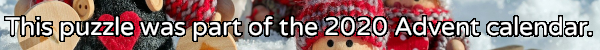mscroggs.co.uk
mscroggs.co.uksubscribe

# Puzzles

## 24 DecemberThere are six ways to put two tokens in a 3 by 3 grid so that the diagonal from the top left to the bottom right is a line of symmetry:
Today's number is the number of ways of placing two tokens in a 29 by 29 grid so that the diagonal from the top left to the bottom right is a line of symmetry.

## 23 December198 is the smallest number that is equal to 11 times the sum of its digits.
Today's number is the smallest number that is equal to 48 times the sum of its digits.

## 14 DecemberThe numbers 33, 404 and 311 contain duplicate digits. The numbers 120, 15 and 312 do not.
How many numbers between 10 and 999 (inclusive) contain no duplicate digits?

## 10 DecemberToday's number is the smallest multiple of 24 whose digits add up to 24.

## 8 DecemberThe residents of Octingham have 8 fingers. Instead of counting in base ten, they count in base eight: the digits of their numbers represent ones, eights, sixty-fours, two-hundred-and-fifty-sixes, etc instead of ones, tens, hundreds, thousands, etc.
For example, a residents of Octingham would say 12, 22 and 52 instead of our usual numbers 10, 18 and 42.
Today's number is what a resident of Octingham would call 11 squared (where the 11 is also written using the Octingham number system).

## 4 DecemberToday's number is a three digit number which is equal to the sum of the cubes of its digits. One less than today's number also has this property.

## 1 DecemberIt is possible to write 325 different numbers using the digits 1, 2, 3, 4, and 5 at most once each (and using no other digits). How many of these numbers are odd?

## 24 DecemberThere are six 3-digit numbers with the property that the sum of their digits is equal to the product of their digits. Today's number is the largest of these numbers.

## Archive

Show me a random puzzle
▼ show ▼
© Matthew Scroggs 2012–2021